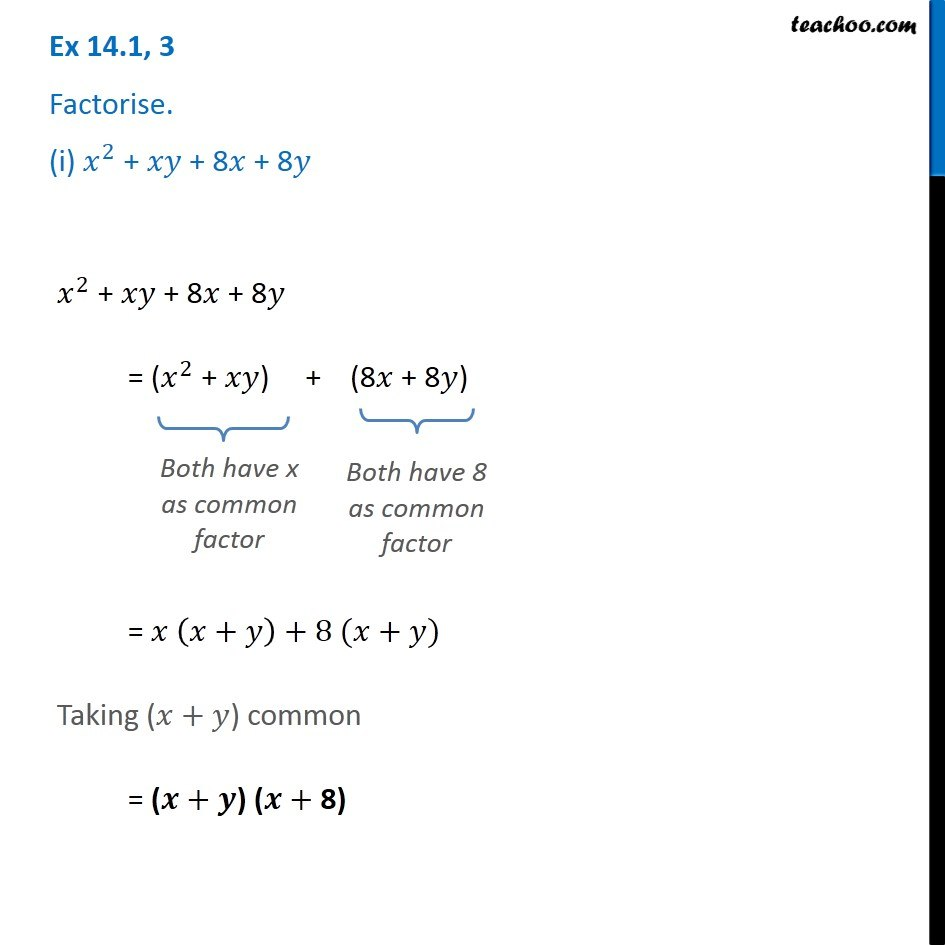1. Chapter 14 Class 8 Factorisation
2. Serial order wise
3. Ex 14.1

Transcript

Ex 14.1, 3 Factorise. (i) 𝑥^2 + 𝑥𝑦 + 8𝑥 + 8𝑦 𝑥^2 + 𝑥𝑦 + 8𝑥 + 8𝑦 = (𝑥^2 + 𝑥𝑦) + (8𝑥 + 8𝑦) Both have x as common factor Both have 8 as common factor = 𝑥 (𝑥+𝑦)+8 (𝑥+𝑦) Taking (𝑥+𝑦) common = (𝒙+𝒚) (𝒙+ 8)

Ex 14.1